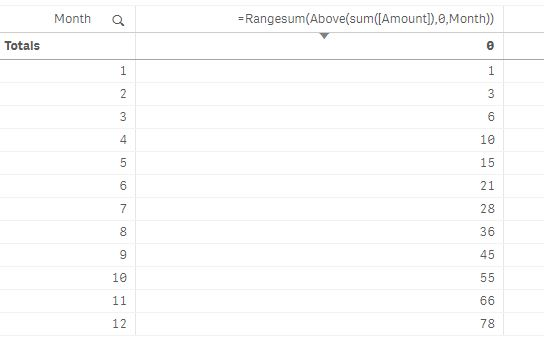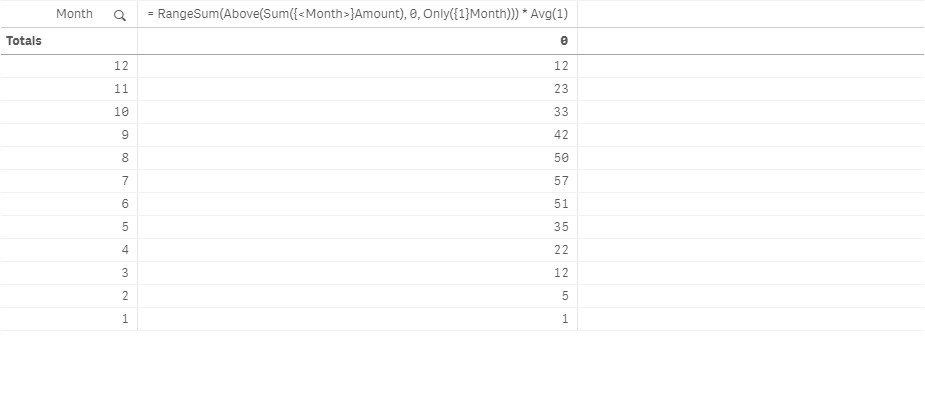# QlikView App Dev

Discussion Board for collaboration related to QlikView App Development.

Announcements
cancel
Showing results for
Did you mean:Contributor III

## YTD calculation that works with filtered date dimension

I have the following sample data:

YearMonthYearMonthAmount
201812018011
201822018022
201832018033
201842018044
201852018055
201862018066
201872018077
201882018088
201892018099
20181020181010
20181120181111
20181220181212

I can calculate the YTD on a straight table like so:However when ever I make a selection on the Month field, the YTD calculation breaks like so (month 6 and 10 are selected):The required result is:

MonthYTD Total
621
1055

Is there a way to write the expression to calculate a YTD total, even when the date dimension in the visualisation is filtered?

I'd like to avoid calculating YTD totals during the script load stage.

Thank you

1 Solution

Accepted SolutionsMVP

Since you are using Qlik Sense, you can def. create a sort independent expression using Aggr() function

Aggr(RangeSum(Above(Sum({<[Month]>}Amount), 0, [Month])), (Month, (NUMERIC)))

The sortable Aggr function is finally here!

6 RepliesMVP

Try this

RangeSum(Above(Sum({<Month>}Amount), 0, Only({1}Month))) * Avg(1)Contributor III
Author

Thank you Sunny. Your suggested expression does work.

Are the following bold elements of the formula necessary?

RangeSum(Above(Sum({<Month>}Amount), 0, Only({1}Month))) * Avg(1)

• Avg(1) should always return 1, so what is the point of multiplying by 1?
• Only() will return the value or null (if there are multiple occurrences). Won't this possibly introduce bugs if NULL is returned?
• {1} doesn't appear to matter, whether I filter on the [Month] field or not.

The following formula appears to work the same: RangeSum(Above(Sum({<[Month]>}Amount), 0, [Month]))

Is it possible to write such a YTD expression which isn't dependent on the sort order?

The suggested formula breaks as soon as the [Month] field's sorted in descending order from 12 to 1 (or any other sort order which isn't strictly ascending). See the example below:MVP

1) Avg(1) will only return for Month which are selected, for anything not selected... Avg(1) = 0. This is what I am using to remove Months which are not selected, because I am ignoring selection in the Month field in the main expression

2) Why will it return Null? Month is a dimension and for every month... you have a possible month (sounds stupid... but it is true, right?)3) I think it should not matter, but I just add it to be safe... feel free to remove it... if it works without Only and {1} after selection alsoMVP

Since you are using Qlik Sense, you can def. create a sort independent expression using Aggr() function

Aggr(RangeSum(Above(Sum({<[Month]>}Amount), 0, [Month])), (Month, (NUMERIC)))

The sortable Aggr function is finally here!Creator III

Use All member filter, so that irrespective of your date selection you would results,

{Starting selection <Dimension1 = {*} >} ({*} for numeric, {"*"} for text)

Or:

{Starting selection <Dimension1 = >}Contributor III
Author

Thank you!

In my previous attempts I got quite close to the solution, but ended up coming up just short due. Thanks for highlighting some of the areas where I misunderstood how the formulas worked.